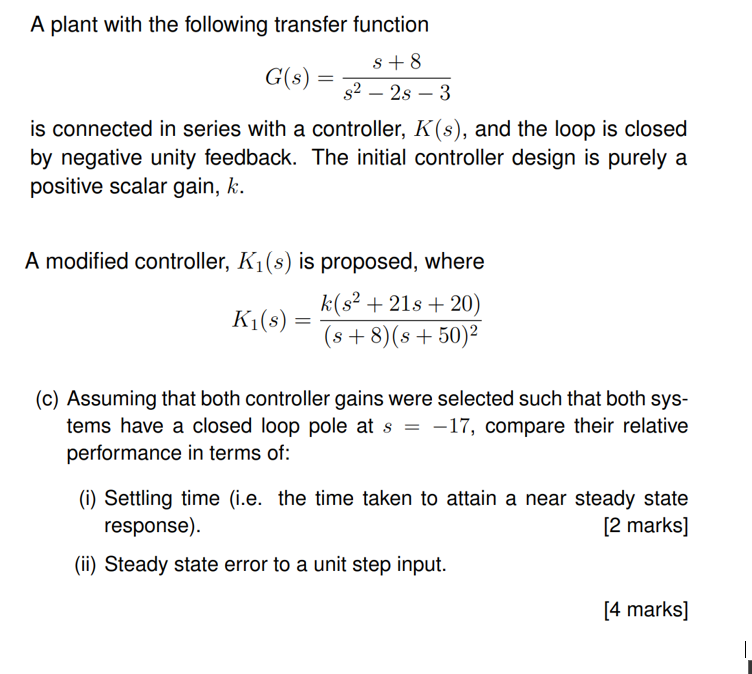Home / Expert Answers / Mechanical Engineering / a-plant-with-the-following-transfer-function-g-s-frac-s-8-s-2-2-s-3-pa925

# (Solved): A plant with the following transfer function $G(s)=\frac{s+8}{s^{2}-2 s-3} ...??????? A plant with the following transfer function \[ G(s)=\frac{s+8}{s^{2}-2 s-3}$ is connected in series with a controller, $$K(s)$$, and the loop is closed by negative unity feedback. The initial controller design is purely a positive scalar gain, $$k$$. A modified controller, $$K_{1}(s)$$ is proposed, where $K_{1}(s)=\frac{k\left(s^{2}+21 s+20\right)}{(s+8)(s+50)^{2}}$ (c) Assuming that both controller gains were selected such that both systems have a closed loop pole at $$s=-17$$, compare their relative performance in terms of: (i) Settling time (i.e. the time taken to attain a near steady state response). [2 marks] (ii) Steady state error to a unit step input.

We have an Answer from Expert